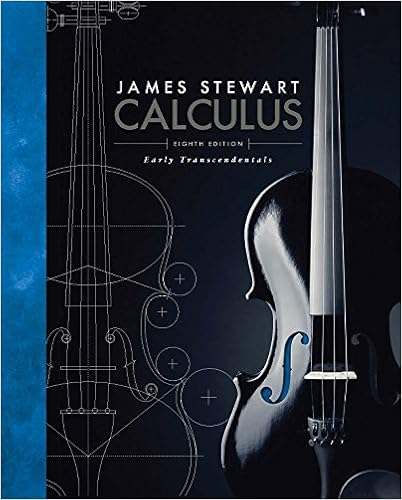# 1013 Tutorial Note 12 - MATH1013 Calculus I(2013 Spring...

• Notes
• 11

This preview shows page 1 - 3 out of 11 pages.

##### We have textbook solutions for you!
The document you are viewing contains questions related to this textbook.The document you are viewing contains questions related to this textbook.
Chapter 7 / Exercise 6
Calculus: Early Transcendentals
StewartExpert Verified
MATH1013 Calculus I (2013 Spring) Tutorial Note 12 ©Henry Cheng @ HKUST May 10, 2013 (Week 14) Page 1 of 15 MATH1013 Calculus I Tutorial Note 12 Topics covered in week 13: 18.Riemann Sums and Definite Integrals (cont’d)19.Fundamental Theorem of Calculus 18.Riemann Sums and Definite Integrals (cont’d)Having seen that the approximation error of Riemann sums can be reduced by partitioning into more subintervals of equal width, we go one step further and try to use a partition of arbitrarily many subintervalsof small widths. This motivates the definition of the definite integralGiven a function ?defined on [?, ?], the definite integralof ?over [?, ?]is defined as the common limit of all its Riemann sumswith respect to any finite partition 𝑃 = {?0, ?1, … , ?𝑁of [?, ?]into ?subintervals, as the width of the largest interval ‖𝑃‖ → 0. In symbols, this means that we define ?(?)????lim‖𝑃‖→0?(𝜔𝑖)(?𝑖− ?𝑖−1)𝑁𝑖=1,whenever this limit exists as the same finite real number for any choice of 𝜔𝑖∈ [?𝑖−1, ?𝑖], and in that case ?is said to be integrable on [?, ?]Note that when 𝒇is integrable on [?, ?], we can focus only on partitions into 𝒏subintervals of equal widthand consider the three particular Riemann sums (left, mid-point and right) that we have seen last week. For example, if we look at right Riemann sumsof ?using a partition of the interval [?, ?]into ?subintervals of equal width𝑛, then the above definition yields lim𝑛→+∞? (? + 𝑖 ⋅? − ??)(? − ??)𝑛𝑖=1=?(?)????;and on the interval [?, ?] = [0, 1]in particular, this formula reduces further to lim𝑛→+∞? (𝑖?)(1?)𝑛𝑖=1=?(?)??10. Geometrically, ∫ ?(?)???is the net areaunder the graph of (the area above ?-axis minusthe area below ???=𝑓(?)𝐴1𝐴2𝐴3𝐴4. }. ?−???
.
##### We have textbook solutions for you!
The document you are viewing contains questions related to this textbook.The document you are viewing contains questions related to this textbook.
Chapter 7 / Exercise 6
Calculus: Early Transcendentals
StewartExpert Verified
MATH1013 Calculus I (2013 Spring) Tutorial Note 12 ©Henry Cheng @ HKUST May 10, 2013 (Week 14) Page 2 of 15 Example 18.4Express each of the following limits as a definite integral, assuming that it exists. (a) lim𝑛→+∞𝑛2+3𝑖(𝑛+𝑖)3𝑛𝑖=1(b) lim𝑛→+∞𝑖3𝑛𝑖=𝑛+1(c) lim𝑛→+∞𝑛cos2𝑖𝜋𝑛𝑛𝑖=1211
(a)Taking a partition of [0, 1]into
1
(b)Taking a partition of [1, 3]into
1
•••